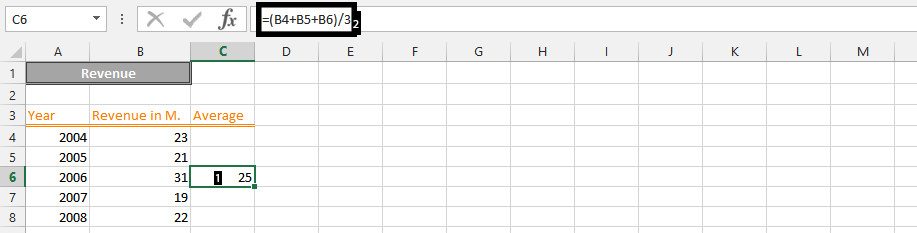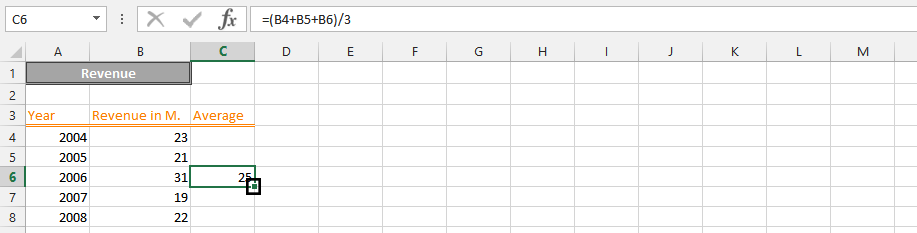### Moving Average In Excel

Calculating a moving average is possible in different ways. It is needed for multiple reasons. However, it begins with having a data that looks something like this:Click on an empty cell (1), type=(B4+B5+B6)/3 (2),and press enter.Note: For the productiveness of performing a moving average, it is preferable that you put the first average value a few rows after the beginning, as you could see in the picture above (1).

If you’d look at the lower right corner (showing on the picture below), you would see on a small square, double click on that small square.Note: This is for calculating the moving average for three years.

In conclusion, the result would look something like this:Moving mean has been calculated in this easy way with Excel.

## Template

```Further reading: